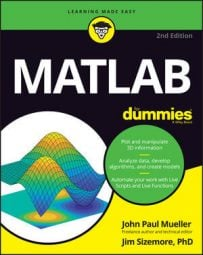##### MATLAB For DummiesGiving an application the capability to perform tasks repetitively with MATLAB is an essential part of creating an application of any complexity. Humans don’t get bored performing a task once. It’s when the task becomes repetitive that true boredom begins to take hold. A computer can perform the same task in precisely the same manner as many times as needed because the computer doesn’t get tired.

## Using the for statement

The for statement performs a given task a specific number of times, unless you interrupt it somehow. Here’s an example of how to use a for loop.

```function [ ] = SimpleFor( Times )
%SimpleFor: Demonstrates the for loop
% Tell the application how many times to say hello!
if nargin < 1
Times = 3;
end
for SayIt = 1:Times
disp(‘Howdy!’)
end
end```

In this case, SimpleFor() accepts a number as input. However, if the user doesn’t provide a number, then SimpleFor() executes the statement three times by default.

Notice how the variable, SayIt, is created and used. The range of 1:Times tells for to keep displaying the message Howdy! the number of times specified by Times. Every time the loop is completed, the value of SayIt increases by 1, until the value of SayIt is equal to Times. At this point, the loop ends.

## Using the while statement

The while statement performs a given task until a condition is satisfied, unless you stop it somehow. This is how to use a while loop in an example.

```function [ ] = SimpleWhile( Times )
%SimpleWhile: Demonstrates the while loop
% Tell the application how many times to say hello!
if nargin < 1
Times = 3;
end
SayIt = 1;
while SayIt <= Times
disp(‘Howdy!’)
SayIt = SayIt + 1;
end
end```

In this example, the function can either accept an input value or execute a default number of times based on whether the user provides an input value for Times. The default is to say “Howdy!” three times.

Notice that the loop code actually begins by initializing SayIt to 1. It then compares the current value of SayIt to Times. When SayIt is greater than Times, the loop ends.

You must manually update the counter variable when using a while loop. Notice the line that adds 1 to SayIt after the call to disp(). If this line of code is missing, the application ends up in an endless loop — meaning that it never wants to end. If you accidentally create an endless loop, you can stop it by pressing Ctrl+C.

## Ending processing using break

It’s possible that a loop will ordinarily execute a certain number of times and then stop without incident. However, when certain conditions are met, the loop may have to end early. The break clause lets you stop the loop early.

This shows how to use the break clause with a while loop, but you can use it precisely the same way with the for loop.

```function [ ] = UsingBreak( Times )
%SimpleWhile: Demonstrates the while loop
% Tell the application how many times to say hello!
% Don’t exceed five times or the application will cut you off!
if nargin < 1
Times = 3;
end
SayIt = 1;
while SayIt <= Times
disp(‘Howdy!’)
SayIt = SayIt + 1;
if SayIt > 5
disp(‘Sorry, too many Howdies’)
break;
end
end
end```

The code executes precisely the same way that the SimpleWhile example works except that this version contains an additional if statement. When someone wants to execute the loop more than five times, the if statement takes effect.

The application displays a message telling the user that the number of Howdies has become excessive and then calls break to end the loop. To see this example in action, type UsingBreak(10) and press Enter in the Command window.

## Ending processing using return

Another way to end a loop is to call return instead of break. The basic idea is the same.

This example shows how to use the return clause with a while loop, but you can use it precisely the same way with the for loop.

```function [ Result ] = UsingBreak( Times )
%SimpleWhile: Demonstrates the while loop
% Tell the application how many times to say hello!
% Don’t exceed five times or the application will cut you off!
if nargin < 1
Times = 3;
end
Result = ‘Success!’;
SayIt = 1;
while SayIt <= Times
disp(‘Howdy!’)
SayIt = SayIt + 1;
if SayIt > 5
disp(‘Sorry, too many Howdies’)
Result = ‘Oops!’;
return;
end
end
end```

Notice that this example returns a Result to the caller. The value of Result is initially set to ‘Success!’. However, when the user gets greedy and asks for too many Howdies, the value changes to ‘Oops!’. To test this example, begin by typing disp(UsingReturn()) and pressing Enter. You see the following output:

```Howdy!
Howdy!
Howdy!
Success!```

In this case, the application meets with success because the user isn’t greedy. Now type disp(UsingReturn(10)) and press Enter. This time the application complains by providing this output:

```Howdy!
Howdy!
Howdy!
Howdy!
Howdy!
Sorry, too many Howdies
Oops!```

## Determining which loop to use

A for loop provides the means to execute a set of tasks a precise number of times. You use the for loop when you know the number of times a task should execute in advance.

A while loop is based on a condition. You use it when you need to execute a series of tasks until the job is finished. However, you don’t know when the task will end until such time as the conditions indicate that the job is done. Because while loops require extra code and additional monitoring, they tend to be slower, so you should use the for loop whenever possible.